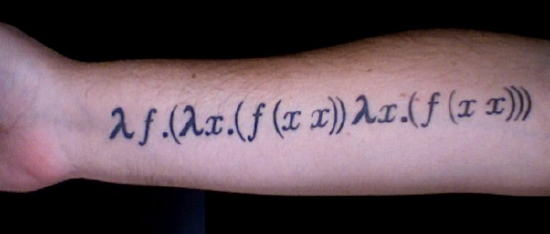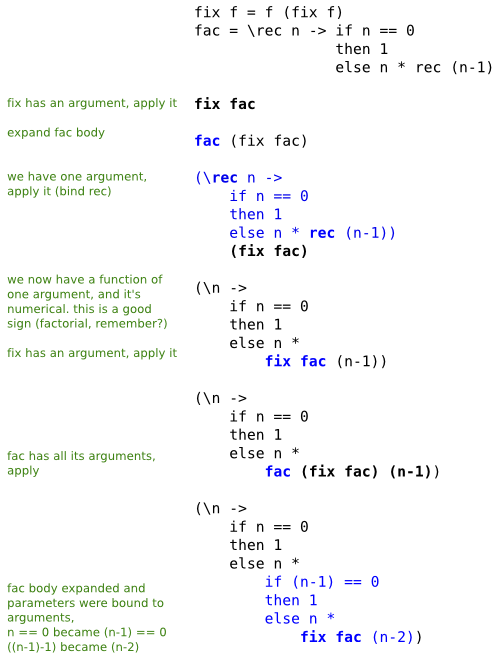## what's in a fixed point?November 9th, 2008

The fixed point is a very intuitive idea that we know from daily life. It's the notion that if something is a certain way, and you do something to change it, it will change. Some things you can go on changing forever by repeating the same action, but other things reach a limit beyond which repeating the action has no effect. Suppose you are going 50km/h in your car and you step on the brake. The car slows down. You step on the brake again, the car slows down again. But eventually the car will have come to a complete stop and stepping on the brake doesn't do anything anymore. The point at which the action has no effect anymore has a scary math name: a fixed point.

It's easy enough to write a function that finds the fixed point of another function f. You apply f to the argument and you get a value, you apply f to that and you get a value, you apply f to that and you get a value etc. And every time you check if the new value is different from the old. (Or even if it's just sufficiently similar, Newton-Raphson says hello.) Here is how it goes:

```fixfinder :: (Eq a) => (a -> a) -> a -> a
fixfinder f arg =
let val = f arg
in if val == arg
then arg
else (fixfinder f val)

{-
*Main> fixfinder sqrt 68
1.0
-}
```

We can try it on a function whose fixed point we know, like the square root. Back in school, did you ever hit the square root key on your calculator repeatedly out of shear boredom? Eventually you'd get 1. (You can also take the square root of 0 and get 0, the second fixed point, but that's not particularly exciting.)

So far so good, now comes the mysterious part. The fixed point idea exists in programming, but in a different guise. In `Data.Function` there is a function called `fix`. It makes a very odd promise: to find the fixed point of a function without using a value. Que? How can you return the fixed point value (leaving aside for the moment the question of how you can even calculate it) if you don't have a single value to begin with? Heck, without an input value you don't even know what type the output is, if f is a polymorphic function.

```fix :: (a -> a) -> a
fix f = f (fix f)

{-
*Main> fix sqrt
*** Exception: stack overflow
-}
```

The function doesn't look promising at all. It applies f, but there is no termination condition. Once you reach the fixed point (if there is one), how do you stop? It obviously can't figure out the square root function.

It turns out that fix is a trick used to perform recursion in languages that don't have a recursion primitive (ie. the compiler reaches an expression containing the name of the function in its own body and doesn't know what to do). The trick is that you write a function expecting among other things, a function argument (fix). Then, at the point in your function where you need to recurse, you call fix. Fix then calls the function back, so it's a sort of mutual recursion. In pure lambda calculus fix is called the y-combinator. The formula is so ridiculously cryptic that some have taken to tattoo it on their arms, obviously given up on remembering.So what do you get out of it? Solely the fact that you get around calling the function within itself, from what I can see. And clearly that's all it does too. How do you prevent the seemingly inevitable infinite loop? You put the termination condition in your semantic function. So you write your recursive function exactly the same, but you give it an extra argument, fix. Then, at the point of recursion, instead of calling the function, you call fix. It's so bizarre that I've written out the evaluation sequence to shed some light on it.

Daredevil that I am, I'm using the factorial function. What's happening here, supposedly, is that fix is computing the fixed point of fac. We can then take that result and apply it to a number, it finds the factorial, it doesn't diverge.What's happening here? It looks like a sort of loop unrolling, which can clearly proceed ad infinitum, were it not for Haskell's lazy nature. And, one can speculate, it's unrolling the recursion exactly the number of times it needs to be expanded (but then again, evaluation with a value already does that), but how could you possibly know that before you have a value to calculate with? It's not actually computing anything, is it? How is this any different from any old recursive function?

What fac really does is take one step toward the factorial. Multiply one factor, then call fix, which calls fac again. Eventually, the termination condition within fac kicks in. But that doesn't mean we've found the fixed point of fac, which is `fac(1) = 1` and `fac(2) = 2`.  In fact, it seems to me we're walking right past these two fixed points, and calling fac one last time with n=0, because how else can fac terminate?

There is a lot more to fixed points, there's even something called a fixed point datatype that my small brain completely fails to comprehend. The most sane description on fix that I found is in the Haskell Book if you want to take a look. I wish you luck.

:: random entries in this category ::

### 2 Responses to "what's in a fixed point?"

1.erik says:

"Back in school, did you ever hit the square root key on your calculator repeatedly out of shear boredom? Eventually you’d get 1"

Aahh good times :D

Rest of this entry is complete abracadabra to me. The scary demon summoning kind.

2.numerodix says:

I know, where are the Ghostbusters when you need them.